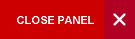Mechanics

Semester 1

This is a calculus-based physics course covering the basic laws and phenomena in mechanics.

Syllabus:
• Scalars and Vectors:
• Scalar and Vector products.
• Vectors and their components.
• Unit vectors.
• Vector algebra in terms of their components.
• Vector Treatment of Motion:
• Position vector and particle trajectory.
• Average and instantaneous acceleration.
• Application to uniform circular motion.
• Derivation of a = -w2r. Relative velocity.
• Work and Kinetic Energy:
• General definition of work.
• Work done by a variable force.
• One-dimensional analysis.
• Interpretation of work as area under graph of F vs. x.
• Proof of Work-Kinetic Theorem.
• Conservation of Energy:
• Conservative Forces.
• General definition of potential energy and examples of its calculation. Mechanical Energy.
• Proof of conservation of Mechanical Energy.
• Non-conservative forces.
• Conservation of total energy.
• System of Particles:
• Centre of mass for systems of particles and extended objects.
• Newton's Second Law for systems of particles and extended objects and consequences.
• Proof of conservation of linear momentum.
• Rotation:
• Description of rotation using θ, w  and α .
• Kinematic equations.
• Kinematic energy of rotation.
• Rotational inertia and its calculation for some symmetrical objects.
• Parallel and Perpendicular Axes Theorem.
• Torque τ = r x F and τ = Iw.
• Work and Torque.
• Rolling:
• Definition of Rolling.
• Rolling as a combination of rotation and translation.
• Rolling as pure rotation  about an instantaneous axis.
• Role of friction in rolling.
• Kinetics and dynamics of rolling.
• Definition of Angular Momentum. Newton's Second Law in angular form.
• Angular momentum for a system of particles.
• Conservation of angular momentum and its application.
• Simple Harmonic Motion:
• Equation of Linear SHM in differential form and solution as x = A sin (ωt + θ).
• Definition of angular SHM in terms of torque and angular displacement.
• Differential equation of motion and its solution.
• Examples such as physical pendulum (and limiting case of simple pendulum) and suspended oscillating disc.
Evaluation:

One 2-hour theory examination paper                                    60%

Two 1-hour in-course tests      (15% each)                              30%

Laboratory Report (Averaged of 6 labs at 10% each)            10%

Learning Objectives:

After completing this course, students should be able to:

•  Distinguish between a scalar quantity and a vector quantity and perform vector algebra.
• Describe and perform calculations related to one and two dimensional motion, as well as rotational and rolling motion, using the basic equations of kinematics.
• Identify, describe and determine mathematically, the cause of linear, rotational and rolling motions, by describing/determining forces, torques, work and energy, impulse and momentum associated with objects undergoing each type of motion.
• Describe mathematically simple harmonic motion and perform simple calculations.
•  Perform and interpret the results of simple experiments and demonstrations of physical principles.
CAPE/A-Level Physics or PHYS0411 and PHYS0412 and PHYS 0421 and PHYS 0422 or CSEC Physics with CAPE/A-Level Maths

Required Textbook:

Halliday, Resnick, and Walker; “Fundamentals of Physics Extended”; 8th Edition, 2007. ISBN 978-0-471-75801-3

Alternative text

Paul A. Tipler and  Gene Mosca,Physics for Scientists and Engineers. 6th Edition, 2007. ISBN-10: 0716789647.

Internet Sources: